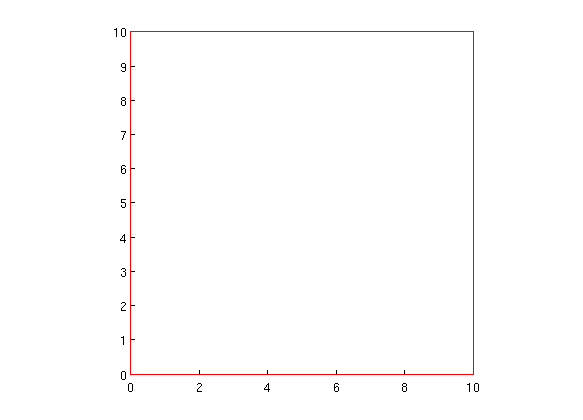## matlab-code-kit

A kit of various, (hopefully) useful pieces of MATLAB code.

My MATLAB codekit features classes for algorithms and data structures like extended kalman filters, rapidly exploring random trees, and collision detectors. For example, this code snippet creates a quad tree of randomly generated points to quickly find all neighbors in some limited range around a point:

``````function test_quad_tree()

q = mcodekit.tree.quad_tree([0 0 10 10], 4, 8);
n = 500;
x = 10.*rand(n,1);
y = 10.*rand(n,1);

for i=1:n
q.insert_point([x(i) y(i)]);
drawnow;
end

j = randi(n);